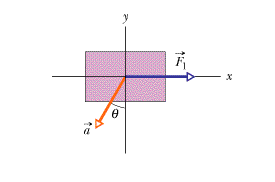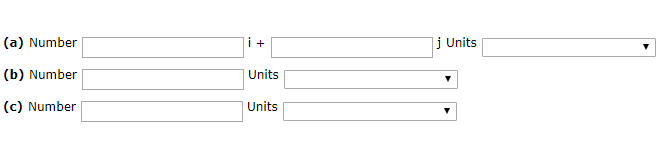# (Solved): There Are Two Forces On The 2.16 Kg Box In The Overhead View Of The Figure But Only One Is Shown. Fo...

There are two forces on the 2.16 kg box in the overhead view of the figure but only one is shown. For F1 = 27.0 N, a = 14.2 m/s^2, and Î¸ = 30.2Â°, find the second force (a) in unit-vector notation and as (b) a magnitude and (c) a direction. (State the direction as a negative angle measured from the +x direction.)(a) Number i + j Units Units (b) Number (c) Number Units

We have an Answer from Expert